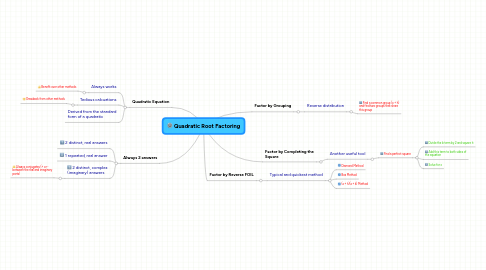Get Started. It's FreeQuadratic Root Factoring### 1.1. Always works

1.1.1. Benefit over other methods

### 1.2. Tedious calcuations

1.2.1. Drawback from other methods

## 2. Factor by Grouping

### 2.1. Reverse distribution

2.1.1. Find a common group (x + h) and find two groups that share this group

## 3. Factor by Reverse FOIL

### 3.1. Typical and quickest method

3.1.1. Diamond Method

3.1.2. Box Method

3.1.3. (x + h)(x + k) Method

### 4.3. 2 distinct, complex (imaginary) answers

4.3.1. Always conjugates (+ or - between the real and imaginary parts)

## 5. Factor by Completing the Square

### 5.1. Another useful tool

5.1.1. Find a perfect square

5.1.1.1. Divide the b term by 2 and square it

5.1.1.2. Add this term to both sides of the equation

5.1.1.3. Solve for x